# YTEP-0028: Alternative Unit Systems¶

## Abstract¶

Created: December 8, 2015 Author: John ZuHone, Nathan Goldbaum, Matthew Turk

This YTEP outlines a plan to support alternative unit systems for yt.

In Progress

## Detailed Description¶

### Background¶

Currently, yt works with a “cgs”-based unit system. That is, all units can be expressible in a set of “base” units, which are reducible to the “centimeter-gram-second” system of units. There is one exception to this rule, that of SI current units which are not reducible to anything within the cgs system, and have a base unit of Amperes.

In the current state of the code, there is minimal support for other unit systems. The extent of this support are the methods for converting unitful quantities (YTArrays, YTQuantities) and units themselves to the SI or “MKS” (meter-kilogram-second) system. These are:

• in_mks: Takes a YTArray or YTQuantity and returns a new one in equivalent MKS base units.
• convert_to_mks: Converts the units of a YTArray or YTQuantity into the equivalent MKS base units.
• get_mks_equivalent: Takes a Unit object and returns the equivalent MKS units.

These methods are useful, but they require the user to convert to MKS “by hand” from the default “cgs” unit system used by yt, within which all calculations are carried out. Some users would prefer to work within the MKS system (or another alternative unit system) which is more appropriate for their datasets and calculations.

This YTEP outlines a proposal for allowing different unit systems to be used in yt. The core of the proposal is to allow this functionality on a per-object basis: namely, changing the unit system at the level of individual datasets, units, and unitful quantities, instead of on a global scale. The advantages of this approach are that it is relatively simple, is easily extendable, and makes only a fairly small number of changes to the fundamental code base.

### The UnitSystem Object¶

Managing different unit systems requires the creation of a new UnitSystem class. A given UnitSystem object will consist of dict-like access to setting and getting default units with the keys corresponding to dimensions, whether strings (e.g., "velocity") or SymPy Symbol objects registered in yt.units.dimensions (e.g., yt.units.dimensions.current_mks). Initialization of a UnitSystem object requires setting the name of the system, as well as a set of base units:

cgs_unit_system = UnitSystem("cgs", "cm", "g", "s")


This will initialize the UnitSystem along with a set of base units. The required arguments are, in order:

• name: The shorthand name for the UnitSystem.
• length_unit: The base length unit for this system.
• mass_unit: The base mass unit for this system.
• time_unit: The base time unit for this system.

The optional arguments are:

• temperature_unit: The base temperature unit for this system. Defaults to "K" (Kelvin).
• angle_unit: The base angular unit for this system. Defaults to "rad" (radians).
• current_mks: The base angular unit for current in an MKS-like system. Defaults to None.

If need be, the base units for temperature, angle, and MKS current can be supplied:

mks_unit_system = UnitSystem("mks", "m", "kg", "s",
temperature_unit="K",
current_mks_unit="A")


The initialization of the UnitSystem will also add it to a unit_system_registry dictionary which may be queried for a given system by its name:

from yt import unit_system_registry
imperial_unit_system = unit_system_registry["imperial"]


Once the UnitSystem exists, new unit defintions for specific dimensions may be added in two ways. The first is to explicitly set a unit for a specific dimension:

from yt.units.import dimensions
mks_unit_system["pressure"] = "Pa"
mks_unit_system[dimensions.energy] = "J"


So, whenever yt asks for the unit corresponding to a given dimensionality (such as in a field definition), the unit specified here will be returned. The second way to add new units to the system is simply by querying for the units for a particular dimension, without having set them previously. The effect of this is to set the units for that specific dimension by deriving them from the base units:

print(mks_unit_system["angular_momentum"]) # We haven't set a unit for this yet!


which will return kg*m**2/s because it will be derived from the base units of m, kg, and s.

Several unit systems will already be supplied for use with yt. They will be:

• "cgs": Centimeters-grams-seconds unit system, with base of (cm, g, s, K, radian). Uses the Gaussian normalization for electromagnetic units.
• "mks": Meters-kilograms-seconds unit system, with base of (m, kg, s, K, radian, A).
• "imperial": Imperial unit system, with base of (mile, lbm, s, R, radian).
• "galactic": “Galactic” unit system, with base of (kpc, Msun, Myr, K, radian).
• "solar": “Solar” unit system, with base of (AU, Mearth, yr, K, radian).
• "planck": Planck natural units (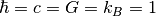), with base of (l_pl, m_pl, t_pl, T_pl, radian).
• "geometrized": Geometrized natural units (), with base of (l_geom, m_geom, t_geom, K, radian).

Users may create new UnitSystem objects on the fly, which will be added to the unit_system_registry automatically as they are created. Both of these will be accessible from the top-level yt module.

#### "code" UnitSystems¶

When a dataset is instantiated, a UnitSystem object corresponding to the code units for that dataset will be created and added to the unit_system_registry, where the name will be the string representation of the Dataset object:

from yt import unit_system_registry, load
sloshing_unit_system = unit_system_registry[str(ds)]


### Unit Systems and Dataset objects¶

The main user-facing interface to the different unit systems will be through the load function. load will take a new keyword argument, unit_system, which will be a string that corresponds to the name identifier for the desired unit system, with a default value of "cgs". The main effect of changing the unit system will be to return all aliased fields and derived fields in the units of the chosen system. For example, to change the units to MKS in a FLASH dataset:

ds = yt.load("GasSloshing/sloshing_nomag2_hdf5_plt_cnt_0100", unit_system="mks")
sp = ds.sphere("c", (100.,"kpc"))
print(sp["density"])
print(sp["flash","dens"])
print(sp["kinetic_energy"])
print(sp["angular_momentum_x"])

[  1.30865584e-23   1.28922012e-23   1.30364287e-23 ...,   1.61943869e-23
1.61525279e-23   1.59566008e-23] kg/m**3

[  1.30865584e-26   1.28922012e-26   1.30364287e-26 ...,   1.61943869e-26
1.61525279e-26   1.59566008e-26] code_mass/code_length**3

[  6.37117204e-13   6.12785535e-13   6.20621019e-13 ...,   3.12205509e-13
3.01537806e-13   3.39879277e-13] Pa

[ -3.97578436e+63  -3.92971077e+63  -3.95375204e+63 ...,   2.39040654e+63
2.39880417e+63   2.44245756e+63] kg*m**2/s


Note that in this example, "density" is an alias to the FLASH field ("flash","dens"), and it has had its units converted to MKS, but the original FLASH field remains in its default code units. "kinetic_energy" and "angular_momentum_x" are derived fields which have also had their units converted.

Another option is to express everything in terms of code units, which may be achieved by setting unit_system="code":

ds = yt.load("IsolatedGalaxy/galaxy0030/galaxy0030", unit_system="code")
sp = ds.sphere("c", (30., "kpc"))
print(sp["density"])
print(sp["kinetic_energy"])
print(sp["angular_momentum_x"])

[    744.93731689     717.57232666     682.97546387 ...,   40881.68359375
57788.68359375  397754.90625   ] code_mass/code_length**3

[    97150.95501972     91893.64007627     85923.44662925 ...,
11686694.21560157  16358988.90006877  79837013.8427877 ] code_mass/(code_length*code_time**2)

[ -1.17917130e-10  -1.05648103e-10  -9.26664470e-11 ...,   2.05149702e-09
2.03607319e-09   6.72304619e-09] code_length**2*code_mass/code_time


Currently, the plan is to have all frontends allow the user to set unit_system in the call to load, but this should be evaluated on a per-frontend basis. For some frontends, it may be more appropriate to set the unit system explicitly, whether to "cgs" or some other system.

### Using UnitSystems in Field Definitions¶

In order for derived fields to take advantage of the different unit systems, it will be necessary to change the units in the field definitions, so that the derived fields may be returned in the units of the system specified when the dataset was loaded.

For example, in setting up the specific angular momentum fields in yt.fields.specific_angular_momentum, we would change the units thus:

def setup_angular_momentum(registry, ftype = "gas", slice_info = None):
unit_system = registry.ds.unit_system
def _specific_angular_momentum_x(field, data):
xv, yv, zv = obtain_velocities(data, ftype)
rv = obtain_rvec(data)
rv = np.rollaxis(rv, 0, len(rv.shape))
rv = data.ds.arr(rv, input_units = data["index", "x"].units)
return yv * rv[...,2] - zv * rv[...,1]

...

function=_specific_angular_momentum_x,
units=unit_system["specific_angular_momentum"],
validators=[ValidateParameter("center")])


Notice that the field definition code itself has not been altered at all except that the units keyword argument to registry.add_field has been changed from cm**2/s to unit_system["specific_angular_momentum"], which will set the units for the field to whatever is appropriate for the unit system associated with the dataset. The unit_system object may be queried with either SymPy symbol objects in yt.units.dimensions or strings corresponding to the variable names of those objects.

This will not be appropriate for all fields–some fields naturally belong in certain units regardless of the underlying system used. In the context of galaxy clusters, "entropy" is an example, which naturally belongs in units of keV*cm**2. Whether or not to change units should be evaluated on a per-field basis.

For users adding their own derived fields, there will be two ways to take advantage of the new unit systems functionality. If derived fields are being created from a dataset using ds.add_field, they can set up the units in a similar way as above:

def _density_squared(field, data):
return data["density"]*data["density"]


If using yt.add_field, however, it will be necessary to set units="auto" in the call to add_field. To provide an extra layer of error handling for this case, a dimensions keyword argument will be added to the DerivedField initialization, which will only be used if units="auto", and will be used to check that the dimensions supplied to add_field and the dimensions of the YTArray in the field definition are the same:

from yt.units.dimensions import temperature

inverse_temp = 1/temperature

def _inv_temperature(field, data):
return 1.0/data["temperature"]
dimensions=inverse_temp)


If one does not supply a dimensions argument when units="auto", or if the dimensions are incompatible, errors will be thrown.

#### Special Handling for Magnetic Fields¶

Making magnetic fields compatible with different unit systems requires special handling. The reason for this is that the units for the magnetic field in the cgs and MKS systems are not reducible to one another. Superficially, it would appear that they are, since the units of the magnetic field in the cgs and MKS system are gauss (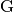) and tesla (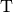), respectively, and numerically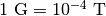. However, if we examine the base units, we find that they have different dimensions: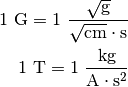It is easier to see the difference between the dimensionality of the magnetic field in the two systems in terms of the definition of the magnetic pressure:where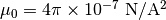is the vacuum permeability. Therefore, in order to handle the different cases of the magnetic field units for the two different systems, it is necessary to have field definitions which can take the different dimensionalities into account.

The most fundamental change which is required will be to change the way aliases are handled for the magnetic field vector fields. Normally, the dataset field and the aliased field will have the same dimensions. For example, in the case of a FLASH dataset, ("flash","magx") and its alias ("gas","magnetic_field_x") will have the same dimensions of magnetic_field_cgs, which are sqrt((mass))/(sqrt((length))*(time)). This is handled by specifying the alias in the known_other_fields atttribute of the FieldInfoContainer like this:

class FLASHFieldInfo(FieldInfoContainer):
known_other_fields = (
...
("magx", (b_units, ["magnetic_field_x"], "B_x")),
("magy", (b_units, ["magnetic_field_y"], "B_y")),
("magz", (b_units, ["magnetic_field_z"], "B_z")),
...
)


Where the alias is the second item in the 3-element tuple after the field name. However, we may want to convert from a cgs unit system to an MKS unit system, which would require changing the dimensions of the alias "magnetic_field_x" (while leaving the units and dimensions of the dataset field "magx" intact). The solution is to remove the alias from known_other_fields and supply a helper function which creates the aliases, taking into account the specified unit system:

class FLASHFieldInfo(FieldInfoContainer):
known_other_fields = (
...
("magx", (b_units, [], "B_x")), # Note the alias has been removed
("magy", (b_units, [], "B_y")),
("magz", (b_units, [], "B_z")),
...
)

def setup_fluid_fields(self):
from yt.fields.magnetic_field import \
setup_magnetic_field_aliases
...
setup_magnetic_field_aliases(self, "flash", ["mag%s" % ax for ax in "xyz"])


Again, this will have to be evaluated on a per-frontend basis as to what is most appropriate for the handling of the magnetic field units. The definitions for other magnetic-related fields such as "magnetic_pressure" and "alfven_speed" will also be modified to ensure that the units are handled properly for the different systems.

### Other Ways to Use the Unit Systems¶

There will be other ways in which unit-aware objects in yt may be converted to a different unit system. they are:

in_base, convert_to_base, get_base_equivalent methods

These three methods, which currently convert unitful quantities and units to the yt base units of cgs (plus Ampere if the dimensionality includes current_mks), will be modified to include a unit_system keyword argument, which will be set to "cgs" by default. The purpose of this keyword argument is to allow switching between different unit systems for YTArrays, YTQuantities, and Unit objects. This keyword argument may be set to a string corresponding to the name of the desired unit system. Some examples:

a = YTArray([1.0, 2.0, 3.0], "km/hr")
print(a.in_base("imperial"))

[ 0.91134442,  1.82268883,  2.73403325] ft/s

b = YTQuantity(12., "g/cm**3")
b.convert_to_base("galactic")
print(b)

1.7730691071344677e+32 Msun/kpc**3

c = YTQuantity(100., "mile/hr")
print(c.units.get_base_equivalent("mks"))

m/s


Alternatively, a Dataset object may be passed as the unit_system argument, which will convert to the base code units of that dataset:

ds = yt.load("IsolatedGalaxy/galaxy0030/galaxy0030")
sp = ds.sphere("c", (30., "kpc"))
print(sp["density"].in_base(ds))

[    744.93731689     717.57232666     682.97546387 ...,   40881.68359375
57788.68359375  397754.90625   ] code_mass/code_length**3


Note that this will only work if the YTArray, YTQuantity, or Unit in question “knows” about those code units, e.g., it is from a data container from that Dataset or was initialized using ds.arr.

A call to in_base or convert_to_base without specifying a unit system will convert to the default “cgs” unit system:

a = YTArray([1.0, 2.0, 3.0], "km/hr")
print(a.in_base())

[ 27.77777778,  55.55555556,  83.33333333] cm/s


which is the current behavior of the code, ensuring backwards-compatibility. The behavior of the cgs and MKS-specific methods (e.g., in_cgs, in_mks, etc.) will not be modified.

Cosmology object

Currently, the Cosmology object returns all quantities in cgs units. The proposed changes will add a new keyword argument, unit_system, which will be a string that corresponds to the name identifier for the desired unit system, with a default value of "cgs".

cosmo = Cosmology(unit_system="galactic")


Alternatively, unit_system may be set to a Dataset object to use the code units of that dataset:

ds = yt.load("IsolatedGalaxy/galaxy0030/galaxy0030")
cosmo = Cosmology(unit_system=ds)


### Physical Constants in the Different Unit Systems¶

Each UnitSystem object will have a constants attribute which can be used to obtain any physical constant in yt.utilities.physical_constants in the base units of that system. For example:

import yt

galactic_units_system = yt.unit_system_registry["galactic"]

G = galactic_units_system.constants.G
clight = galactic_units_system.constants.clight
mp = galactic_units.system.constants.mp

print(G)
print(clight)
print(mp)

4.498205661165364e-12 kpc**3/(Msun*Myr**2)

306.6013938381177 kpc/Myr

8.417430465502256e-58 Msun


### Notifying the Community¶

The community will be notified about this feature enhancement via the mailing list and appropriate social media accounts. Appropriate documentation of this feature will be added.

## Backwards Compatibility¶

Since the base unit system for all yt units will remain cgs, and the unit_system keyword will always default to "cgs" for loading datasets, setting up Cosmology objects, and unit conversions of arrays, the changes as proposed are fully backwards-compatible.

## Alternatives¶

The only alternative discussed up to this point was to set the unit system globally for a given yt session using the configuration system. The system proposed here allows for more fine-grained control at the level of individual objects, e.g. Dataset, YTArray, and Cosmology objects, which should be sufficient for most (if not all) purposes. Another option is to make the default base units themselves configurable. This is disfavored since it does not appear to add additional functionality beyond the currently proposed scheme, and would result in more widespread changes to the code base.